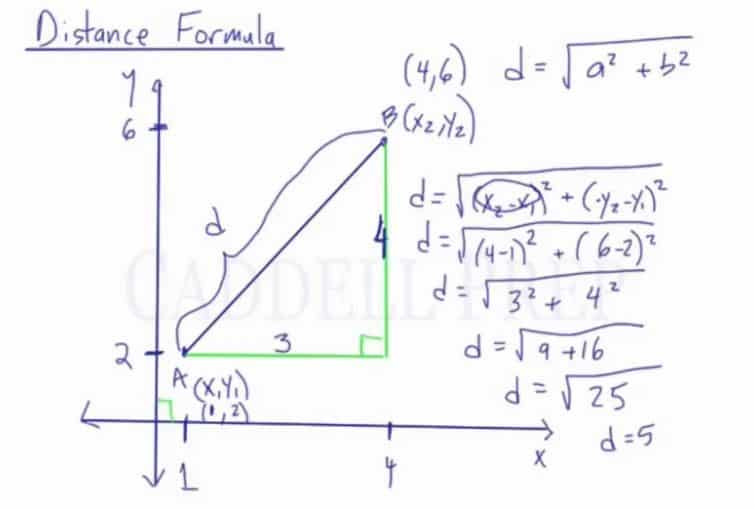Distance Formula

In this video, we are going to look at the distance formula. The distance formula is used to find the distance between two points, so in this case, the distance from A to B. The video lesson above will cover a midpoint formula example and show how the midpoint formula is related to Pythagorean Theorem.

What is the Distance Formula?

Distance Formula:$d=\sqrt{(x_2-x_1)^2+(y_2-y_1)^2}$

For example:
If point A was (1,2) and point B was (4,6), then we have to find the distance between the two points.$d=\sqrt{(4-1)^2+(6-2)^2}$$d=\sqrt{(3)^2+(4)^2}$$d=\sqrt{9+16}$$d=\sqrt{25}$$d=5$

If you don’t want to memorize the formula, then there is another way to find the distance between the two points. Draw a line from the lower point parallel to the x-axis, and a line from the higher point parallel to the y-axis, then a right triangle will be formed. Then, we can use the Pythagorean theorem to solve for the distance.

The distance of one leg is the difference in the x’s. 4-1=3 so that side of the triangle is 3. The distance of the other leg is the difference in the y’s. 6-2=4 so that side of the triangle is 4. Now we can use the Pythagorean theorem. The distance formula is actually based off of that:$d=\sqrt{a^2+b^2}$

If we solved using the Pythagorean theorem, then:$d^2=3^2+4^2$$d^2=9+16$$d^2=25$$\sqrt{d^2}=\sqrt{25}$$d=5$The distance from point A to point B is found using the distance formula and by using Pythagorean Theorem.

Video-Lesson Transcript

In this lesson, let’s discuss the distance formula.

The distance formula is used to find the distance between two points.

In this case, distance from point$A$ to point$B$.

We have coordinates$(x_1, y_1)$ for point$A$.

And point$B$ has coordinates$(x_2, y_2)$.

Distance formula is$d = \sqrt{(x_2 - x_1)^2 + (y_2 - y_1)^2}$

You can memorize this formula and find the distance between any two points.

Let’s have an example.

Point$A$ has coordinates$(1, 2)$. Point$B$ has coordinates$(4, 6)$. Let’s find the distance between these two points.

Distance formula is$d = \sqrt{(x_2 - x_1)^2 + (y_2 - y_1)^2}$$d = \sqrt{(4 - 1)^2 + (6 - 2)^2}$$d = \sqrt{(3)^2 + (4)^2}$$d = \sqrt{9 + 16}$$d = \sqrt{25}$

Now, we have$d = 5$

This means that the distance between point$A$ and point$B$ is$5$.

If you don’t want to memorize the formula, there’s another way to find the distance between the two points.

It’s very similar to the formula.

Let’s draw a triangle using the line from$A$ to point$B$.

Make sure to make to form a triangle with a$90^{\circ}$ angle.

Then, we can use the Pythagorean theorem.

The distance of the horizontal leg is the difference of the two$x$-coordinates. Let’s call it$a$.$a = 4 - 1$$a = 3$

Then, the distance of the vertical leg is the difference of the two$y$-coordinates. Let’s call this leg$b$.$b = 6 - 2$$b = 4$

Now, take a look at the Pythagorean theorem.$a^2 + b^2 = c^2$

The distance formula is actually based on this.

So, let’s solve this using Pythagorean theorem$d^2 = 3^2 + 4^2$$d^2 = 9 + 16$$d^2 = 25$

Then, let’s get the squareroot of$d$ to get the answer.$\sqrt{d^2} = \sqrt{25}$$d = 5$

So, we got the same answer as the first one.

So now, you could memorize the distance formula or you could draw a triangle and use the Pythagorean theorem.